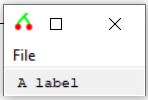# SOB – Object – Labels

This executable supports a label SOB.

In many ways it is like a button:

• it can respond to mouse clicks,
• you can get/set its displayed text
• you can get/set its colour

The following script will a simple label and respond to mouse clicks by changing its colour and its text (title).

`newscript`
`dim myWindow = sob (0, "add","window","Hello World")`
`function ClickMyWindow ( sobid )`
`sob ( myWindow, "delete")`
`quit`
`End function`
`sob ( myWindow , "ON" , "CLICK" , "ClickMyWindow" )`
`dim myMenuBar = sob (myWindow , "add" , "menu" , "bar" )`
`dim myMenuFile = sob (myMenuBar , "add" , "menu" , "Horizontal" , "File" )`
`dim myFileExit = sob ( myMenuFile , "add" , "menu" , "Vertical" , "Exit" )`
`sob ( myFileExit , "ON" , "CLICK" , "ClickMyWindow")`
`' --------------------`
`dim myContainer = sob ( myWindow , "add" , "CONTAINER" , "COLUMN" , 3)`

`' --------------------`
`dim myLabel = sob ( myContainer, "add" , "LABEL" , " A label " ) `
`function Press ( sobid )`

`dim newTitle `
`if ( sob(sobid , "GET", "RGB") == rgb(255,255,0) ) then `
`sob(sobid , "SET", "RGB" , rgb( 0,150,255) )`
`newTitle ="Now I am blue"`
`ELSE`
`sob(sobid , "SET", "RGB" , rgb(255,255,0) )`
`newTitle ="Now I am yellow"`
`End IF `
`sob(sobid, "SET","TITLE", newTitle)`
`End function`

`sob ( myLabel , "ON" , "CLICK" , "Press")`
`sob ( myWindow , "SET" , "SHRINK" )`

The following is a snapshot of the GUI .##### Functional specifications for label related SOB commands:
`SOB ( _`
`<Parent: number expression> ,_`
`"ADD" , _"LABEL" , _``"<TITLE: string expression>" , _`
`)`